Final Project – Knockdown warning indicator

Coming together (in a New York hotel room)Anemometer – wind speed indicator.

I 3D printed the part from a model I made in Rhino, and mounted it on a 12v DC motor.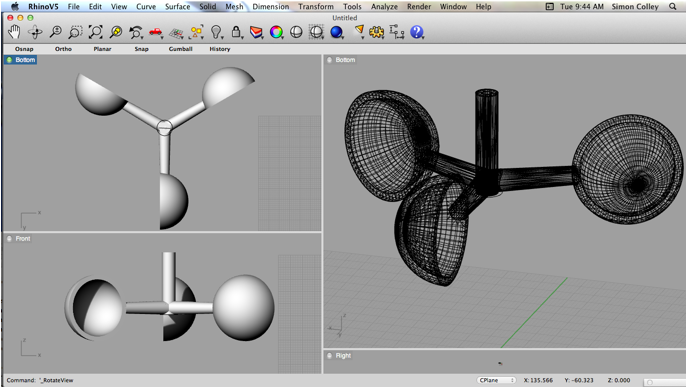Inclinometer – step response based on TxRx, using a curved piece of hose and some copper strips.  I had initially planned on filling the ends with hot glue, but this slowed down the water movement considerably and thus reduced the effectiveness of the sensor, so I only partially filled them.  I mounted the tube on a piece of birch ply I cut out using the CNC 3-axis mill in the hobby shop.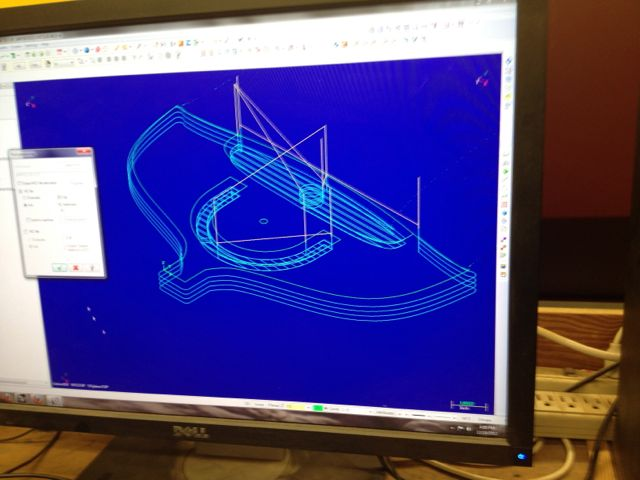I routed the edges of the milled piece using a table router, sanded by hand, and finished using a satin urethane gel.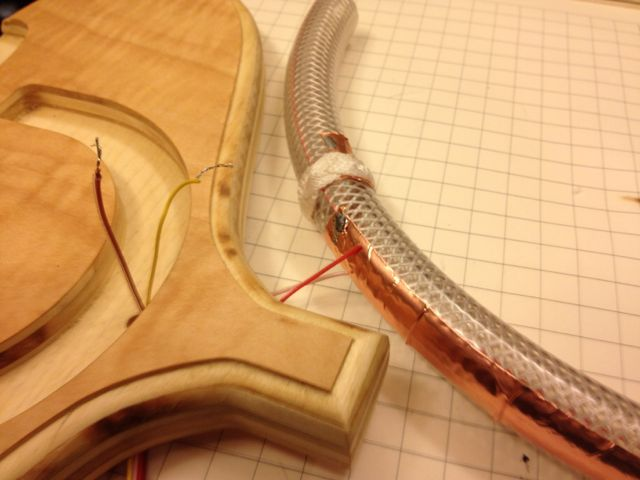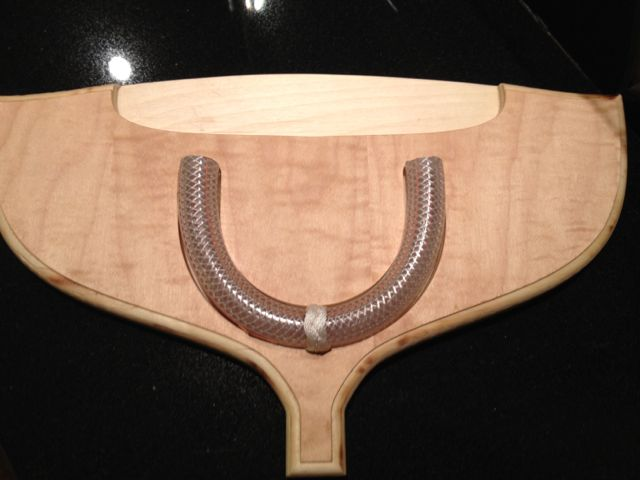Hooked this up to the hello.txrx.45 board and it worked beautifully.

I initially planned on using a Fabduino and running an LCD, but found myself spending a lot of time troubleshooting, so I decided to use the original board I designed for use with an accelerometer.  I figured it had 3 pins connected to a header, which also carried VCC and GND, so that should work.  It also already carried labeled warning LEDs for the three ranges of squall condidtions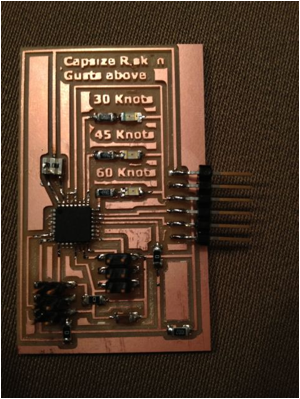Made up an acrylic housing using the laser cutter.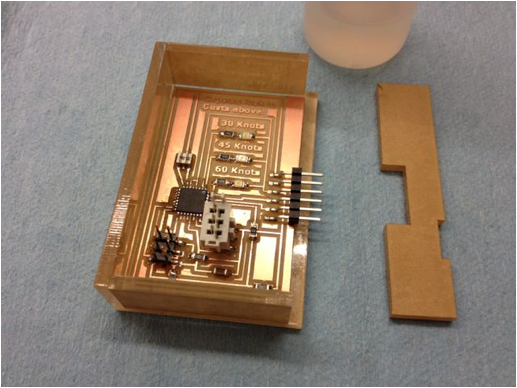Now it all hinges on the code,  which I’m still troubleshooting:

// Knockdown

// Cobbled together by Simon Colley

// This is meant to run on an ATMEL ATMega328

#include <avr/io.h>

#include <util/delay.h>

#define output(directions,pin) (directions |= pin) // set port direction for output //this block is same as neil's, except for the define inputs line , which I don't know whether I need

#define input(directions,pin) (directions &= (~pin)) // set port direction for input

#define set(port,pin) (port |= pin) // set port pin

#define clear(port,pin) (port &= (~pin)) // clear port pin

#define pin_test(pins,pin) (pins & pin) // test for port pin

#define bit_test(byte,bit) (byte & (1 << bit)) // test for bit set

#define bit_delay_time 100 // bit delay for 9600 with overhead

#define bit_delay() _delay_us(bit_delay_time) // RS232 bit delay

#define half_bit_delay() _delay_us(bit_delay_time/2) // RS232 half bit delay

#define settle_delay() _delay_us(100) // settle delay

#define char_delay() _delay_ms(10) // char delay

#define nloop 100 // loops to accumulate

#define serial_port PORTD // changed from Neil's, to suit an ATMEGA

#define serial_direction DDRD

#define serial_pin_out (1 << PD1)

#define transmit_port PORTC

#define transmit_direction DDRC

#define transmit_pin (1 << PC1)

#define anemometer_port PORTC //suspect this will need to be changed to a different port - can I have inputs and outputs at the same time?

#define anemometer_direction DDRC

#define anemometer1 (1 << PC0)

#define anemometer_pins PINC

//outputs = three LEDs

#define led_port PORTD

#define led_direction DDRD

#define sixty (1 << PD2)

#define fortyfive (1 << PD3)

#define thirty (1 << PD4)

#define led_delay() _delay_ms(100) // LED delay

#define PWM_delay() _delay_ms(1000) // PWM delay

void put_char(volatile unsigned char *port, unsigned char pin, char txchar) {

//

// This is all straight from Neil's hello.txrx.45 code

//

// send character in txchar on port pin

//    assumes line driver (inverts bits)

//

// start bit

//

clear(*port,pin);

bit_delay();

//

// unrolled loop to write data bits

//

if bit_test(txchar,0)

set(*port,pin);

else

clear(*port,pin);

bit_delay();

if bit_test(txchar,1)

set(*port,pin);

else

clear(*port,pin);

bit_delay();

if bit_test(txchar,2)

set(*port,pin);

else

clear(*port,pin);

bit_delay();

if bit_test(txchar,3)

set(*port,pin);

else

clear(*port,pin);

bit_delay();

if bit_test(txchar,4)

set(*port,pin);

else

clear(*port,pin);

bit_delay();

if bit_test(txchar,5)

set(*port,pin);

else

clear(*port,pin);

bit_delay();

if bit_test(txchar,6)

set(*port,pin);

else

clear(*port,pin);

bit_delay();

if bit_test(txchar,7)

set(*port,pin);

else

clear(*port,pin);

bit_delay();

//

// stop bit

//

set(*port,pin);

bit_delay();

//

// char delay

//

bit_delay();

}

int main(void) {

//

// main

//

static unsigned char count;

static uint16_t up,down;

//

// set clock divider to /1

//

CLKPR = (1 << CLKPCE);

CLKPR = (0 << CLKPS3) | (0 << CLKPS2) | (0 << CLKPS1) | (0 << CLKPS0);

//

// initialize output pins

//

set(serial_port, serial_pin_out);

output(serial_direction, serial_pin_out);

clear(transmit_port, transmit_pin);

output(transmit_direction, transmit_pin);

//

// init A/D

//

ADMUX = (0 << REFS2) | (0 << REFS1) | (0 << REFS0) // Vcc ref

| (0 << ADLAR) // right adjust

| (0 << MUX3) | (0 << MUX2) | (1 << MUX1) | (1 << MUX0); // PC0

ADCSRA = (1 << ADEN) // enable

| (1 << ADPS2) | (1 << ADPS1) | (1 << ADPS0); // prescaler /128

//

//initialize the anemometer - This is stuff I've added.

clear(anemometer_port, anemometer1);

input(anemometer_direction, anemometer1);

//

// initialize the LEDs - added this also

clear(led_port, sixty | fortyfive | thirty); // | is bitwise operation, || is logical OR

output(led_direction, sixty | fortyfive | thirty);

set(led_port, sixty | fortyfive | thirty);

//

// main loop

//

while (1) {

//

// accumulate

//

up = 0;

down = 0;

for (count = 0; count < nloop; ++count) {

//

// settle, charge

//

settle_delay();

set(transmit_port, transmit_pin);

//

// initiate conversion

//

//

// wait for completion

//

;

//

// save result

//

//

// settle, discharge

//

settle_delay();

clear(transmit_port, transmit_pin);

//

// initiate conversion

//

//

// wait for completion

//

;

//

// save result

//

}

//

// send framing

//

put_char(&serial_port, serial_pin_out, 1);

char_delay();

put_char(&serial_port, serial_pin_out, 2);

char_delay();

put_char(&serial_port, serial_pin_out, 3);

char_delay();

put_char(&serial_port, serial_pin_out, 4);

//

// send result

//

put_char(&serial_port, serial_pin_out, (up & 255));

char_delay();

put_char(&serial_port, serial_pin_out, ((up >> 8) & 255));

char_delay();

put_char(&serial_port, serial_pin_out, (down & 255));

char_delay();

put_char(&serial_port, serial_pin_out, ((down >> 8) & 255));

char_delay();

}

// get anemometer values

//

//think

if wind = 10 and angle = 5

or if wind = 12 and angle = 10

or if wind = 16 and angle = 15

or if wind = 18 and angle = 20

or if wind = 20 and angle = 25

or if wind = 22 and angle = 30

then

clear(led_port,sixty); //otherwise turn it on!

PWM_delay();

set(led_port,sixty); //turn off

else if wind = 15 and angle = 5

or if wind = 20 and angle = 10

or if wind = 24 and angle = 15

or if wind = 27 and angle = 20

or if wind = 30 and angle = 25

or if wind = 32 and angle = 30

then

clear(led_port,fortyfive); //otherwise turn it on!

PWM_delay();

set(led_port,fortyfive); //turn off

else if wind = 15 and angle = 5

or if wind = 20 and angle = 10

or if wind = 24 and angle = 15

or if wind = 27 and angle = 20

or if wind = 30 and angle = 25

or if wind = 32 and angle = 30

then

clear(led_port,thirty); //otherwise turn it on!

PWM_delay();

set(led_port,thirty); //turn off

}